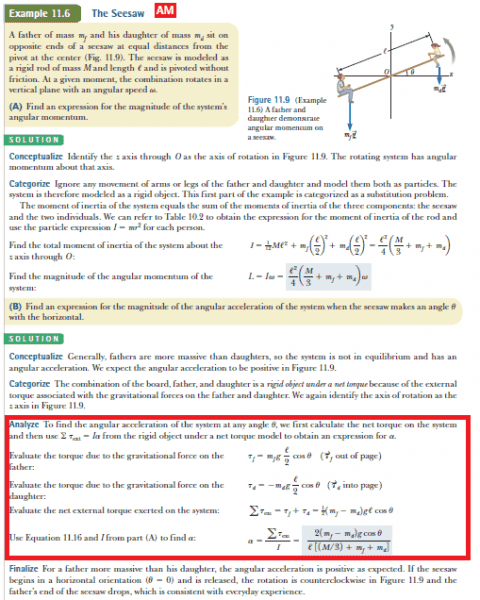# Angular Momentum: Rotating Object

## Homework Statement

I'm trying to understand an example from my textbook about angular momentum. This is the example given:For the part in red: I don't understand where the cosine theta term came from. When you're calculating the magnitudes of torques, don't you just use FRsin(theta)? If someone could clear that up for me, it would be great! Thank you.haruspex
Homework Helper
Gold Member
I don't understand where the cosine theta term came from. When you're calculating the magnitudes of torques, don't you just use FRsin(theta)?
If the theta is the angle between the force vector and the distance vector, yes. But in the diagram, the angle between the vectors is the angle between the vertical and the seesaw. Theta is the angle between the horizontal and the seesaw.

Ok. I think I get it; the cosine is used to get the moment arm in this case, correct?

haruspex
Homework Helper
Gold Member
Ok. I think I get it; the cosine is used to get the moment arm in this case, correct?
That's one way to look at it. There are at least 3 ways, leading to the same answer:
- distance cos (theta) = moment arm.
- force cos (theta) = component of force perpendicular to distance
- vector product of force and distance = force * distance * sin(90-theta)

•Speedking96
Ok, I understand. Thank you very much.